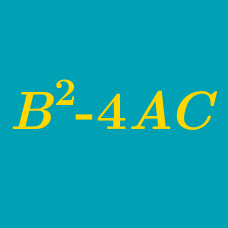Algebra

# Finding the Discriminant of a Quadratic

What is the discriminant of the quadratic polynomial $4x^2+11x+1$?

If the discriminant of the quadratic $2x^2 + ax + 72$ is 0, what is $a$ if $a > 0$?

If $a$ is a nonzero real number, what is the discriminant of the quadratic polynomial $ax^2-8x-\frac{1}{a}?$

If $a$ and $b$ are real numbers such that the discriminant of the quadratic polynomial $\frac{11}{2}x^2+2(a+11b)x+8ab$ equals $0$, what is $\frac{a}{b}$?

What is the discriminant of the quadratic $x^2 - 10x + 2$ ?

×